﻿ Spectrometry and Ellipsometry Analysis -- Redeeming Time DesignsTHIN FILM ANALYSIS USING SPECTROMETRY AND ELLIPSOMETRY

I.  OBJECTIVE 1:

I.1.  Spectrophotometer

A spectrophotometer varies the wavelength of a collimated beam of light over a certain range.  The beam of light is transmitted through a sample to a detector to measure percent transmission versus wavelength.  To do this, first a baseline measurement must be made, which includes transmitting the beam through the air (no sample) or through a "dummy" sample to allow percent transmission to be determined for a sample afterward.  One use for the spectrophotometer is to measure the absorption frequencies of solid, liquid, or gas dielectric material.  These tests are valuable for determining the chemical make-up and properties of materials.

If the wavelengths of the spectrophotometer are "mono-chromatic enough", at each interval in the wavelength scan, then thin film studies can be performed.  The frequency or spectral bandwidth is a measure of how mono-chromatic a light source is .  The smaller the bandwidth, the closer the light source is to perfect mono-chromatic light, and the larger the coherence length.  The coherence length is a measure of the possible path difference two parts of the same wave front can take and still interfere when recombined.  A wave front passing through a thin film is divided (reflected) at the two interfaces (surface of the film).  The reflected wave fronts interfere, causing a variation of the intensity of transmitted light as the wavelength varies.  The way the transmitted intensity varies with wavelength allows for the determination of the thickness or the index of refraction of the film.

The Cary 5 Spectrophotometer, used in this study, uses a filter wheel and then three slit devices to produce very mono-chromatic light from a mercury lamp light source.  After the second slit, the wavelength has a spectral bandwidth of 2 nm (λ � 2 nm).  The third slit is adjustable and can reduce the bandwidth to a greater degree.  The Cary 5 has a total wavelength range of 175 to 3,300 nanometers, through the use of two light sources and five unique filters in conjunction with the three slit devices.

An example of a transmission test on a transparent thin film is shown in Figure 1.  Figure 1 shows that above a wavelength of 400 nm, over 90% of the radiation is transmitted.  But there are sinusoidal variations.  The maximum and minimum values of these variations corresponds to wavelengths given by the following equation: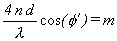1

where m must be an integer, depending on the environment on each side of the film, maxima and minima correspond of m being odd or even.  n is the index of refraction and d is the thickness of film.  φ' is the angle of the wave front in the film.  Equation 1 indicates that a maxima or minima occurs when the optical path difference, caused by travel through the film, is equal to an integral number of half wavelengths (λ/2).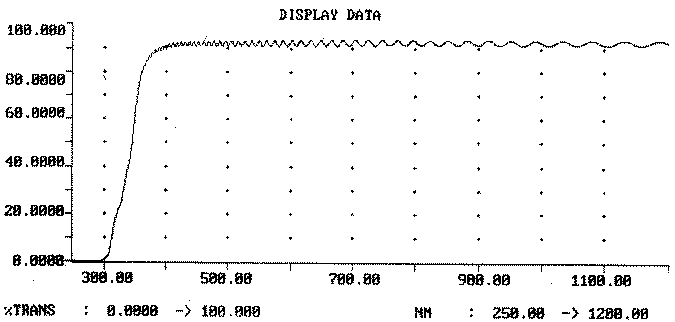FIGURE 1:  Example of spectrophotometer trace of a transparent, thin film sample.

By determining the wavelengths at maxima and minima, the product of n*d can be determined.  However, neither n nor d can be determined independently.  This is a major drawback to this method because either n or d must be known.  In semiconductor work, as in this work, it is n that is usually assumed from literature or other experimentation.  However, the value of n can vary due to the nature of the manufacture of the film.  This not only can lead to significant errors in d but also neglects vital information to be gleamed from the value of n.  (For example, a lower than expected value of n may indicate a porous film.)  Both n and d could be evaluated from several maxima (and/or minima) if the dispersive equation for the index of refraction of the film is known to be of a definite form.  However, dispersive equation may also vary with the quality of the film.

In order to determine the n and d values for transparent films on top of a reflecting (non-transmitting) surface, a special adaptive fixture was designed and built for the Cary 5 Spectrophotometer, as shown in Figure 2.  The fixture reflects the beam of light on a front surface (probably silver) mirror up to the sample.  The light strikes the sample at an incidence angle of 30o.  Then the light is reflected on a second front surface mirror to the detector.  The fixture was meticulously machined to assure precise angles.  The fixture was designed and produced to hold the sample parallel to sample holder and the mirrors set, at high tolerance, 30o to the sample holder.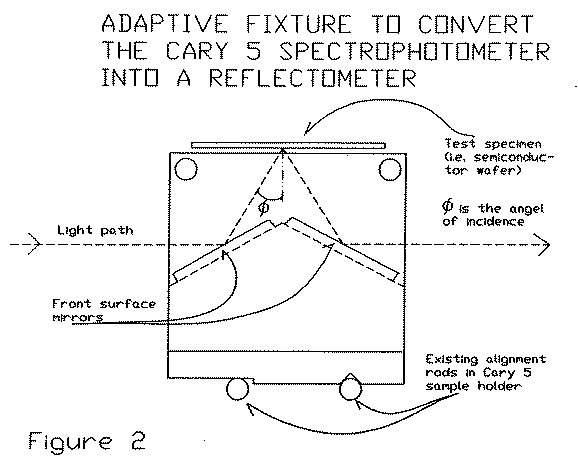FIGURE 2:  Adaptive fixture to convert the Cary 5 Spectrophotometer into a reflectometer.

The concerns with this adaptation include:   the fact that the light beam diverges (as shown in Figure 3) and is not designed to travel the extra distance this fixture imposes, and the fact that reflection on the two mirrors alter the nature of light.  These concerns are alleviated by the fact that data taken in reference to a primary baseline trace.  To do a baseline measurement at the proper wavelength range, the fixture is placed on the sample holder and a "bare" substrate is placed on the fixture.  The reflected intensity of subsequent sample traces is recorded as the percentage of the intensity which reached the detector when the baseline was taken.  Therefore, abnormalities caused by the fixture will be factored out.  However, one factor unaccounted for is the fact that there will be a native oxide on the substrate used for the baseline.  However, with silicon for example, the native oxide (SiO2) will be so thin that it will not cause gross variations.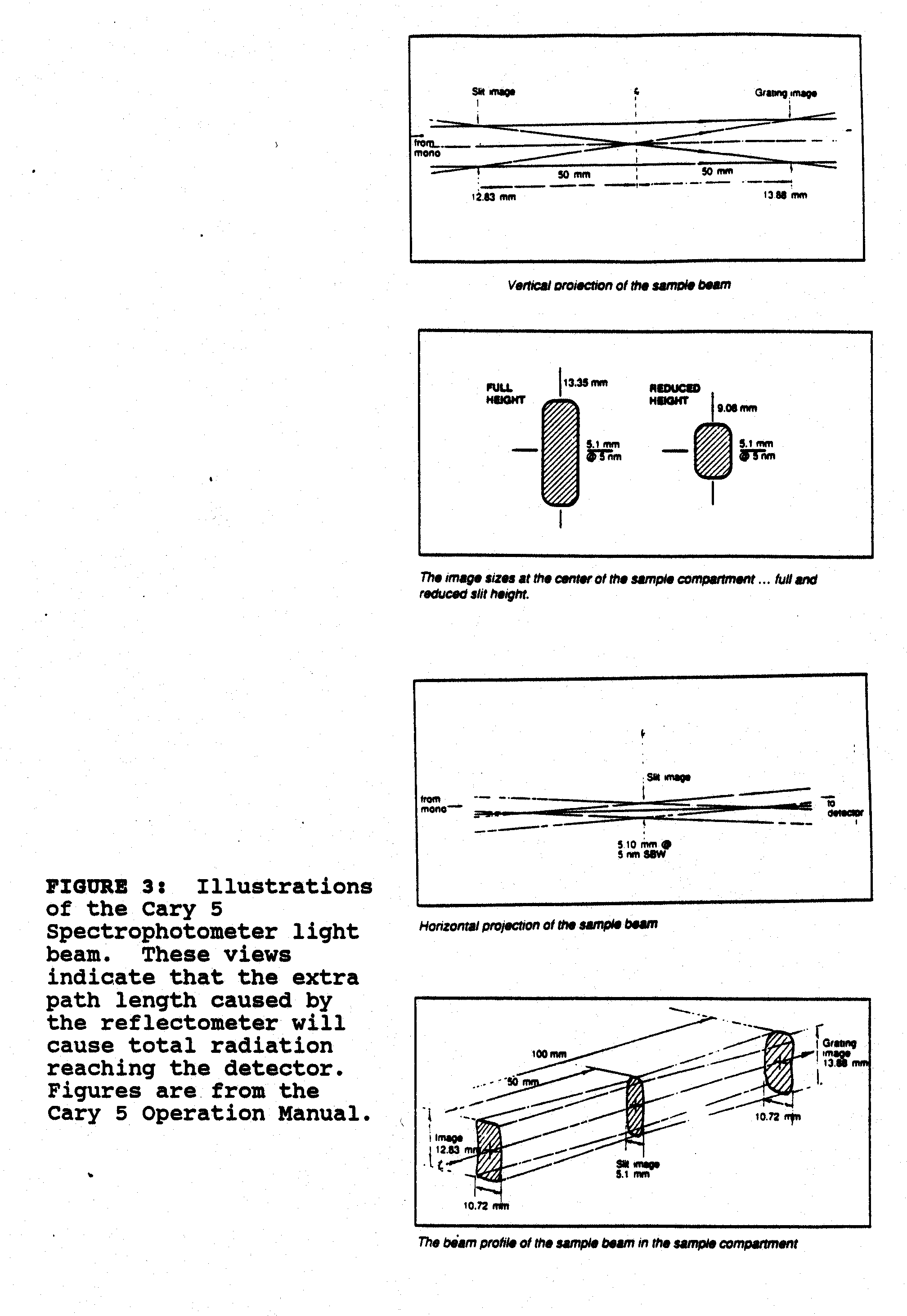I.3  Sample Preparation:

The author was fortunate to be granted access to the cleanroom, to be gifted with seven 3" diameter silicon wafers, and most notably, to receive the assistance of David Price.  All eight wafers were given an RCA clean to remove ionic and organic contaminants as well as the native oxides.  (The description of the RCA clean procedure is included in the Appendix.)

Five of the wafers were wet oxidized.  This involves placing the cleaned wafers into a furnace.  The furnace seals the wafers in a fused silica chamber heated by elements in its wall.  Oxygen and hydrogen are introduced into the chamber at high pressure, and release heat while turning into steam.  The steam diffuses into the silicon at a much faster rate than oxygen does, due to ability of hydrogen to diffuse into silicon rapidly.   Therefore, wet oxidation (with steam) is used when thick oxides are required.  However, the oxide produced is generally more porous and of lesser quality than oxide produced by dry oxidation (no steam).  Figure 4 shows the rates versus temperature for wet and dry oxidation.  Hydrogen and oxygen are introduced in a ratio of 2 : 1 and produce the following chemical reaction with the silicon:

Si  +  H2O    SiO2  +  2H2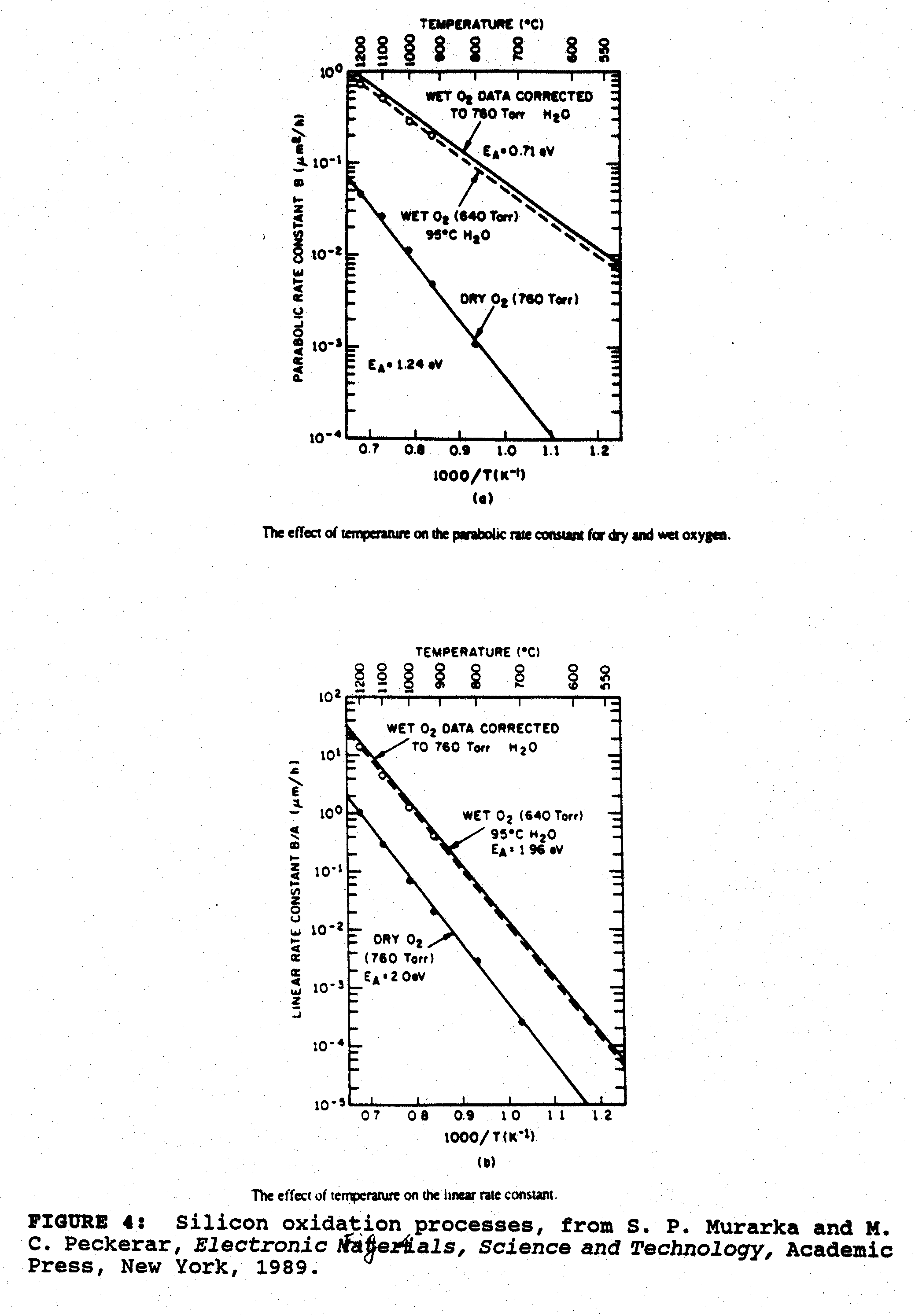The parameters for the oxidation were designed to produce an oxide thickness of one micron.  The parameters include:

Ramp up:      20 minutes

Preanneal:     20 minutes

Oxidation:     17 minutes  (Steam at 1,000 oC)

Postanneal:    40 minutes

Ramp Down: 40 minutes

Silicon nitride, which is typically used as a high temperature mask, was deposited on one wafer (after RCA clean).  The nitride was deposited by chemical vapor deposition at a temperature of 800 oC and a NH3 flow-rate of 120 liters/minutes.  The nitride was deposited for five minutes.

After the RCA clean, wax was applied to the five wafers which were oxidized and to the wafer which was deposited with nitride.  The concept was that the wax prevents oxidation or nitride deposit.  Therefore, after these two procedures, the wax was removed (by hot trichloroethylene) to reveal "bare" silicon surfaces.  Then a machine called a Dektak, which is basically a profilometer, uses a mechanical stylus to slide along the "bare" silicon surface into the surface covered with the oxide or the nitride.  The machine measures the vertical displacement of the stylus when this occurs.  This measurement is inaccurate, but it ball-parks the thickness, which is useful because the ellipsometer returns several possible thickness values, equally and significantly separated.

The above procedure has been described because during assistance in the cleanroom, the wax was applied in typical manner, which was inappropriate for this work.   A thin "X" was made with the wax over the entire wafer, as shown in Figure 5.  At first it was thought that this unfortunate action was terminal because there was no way to position the wafer on the fixture and totally avoid radiation of the "X" region by the spectrophotometer beam.  However, not wanting to abuse the generosity of the cleanroom personnel, it was considered that any light incident on the "X" region the same as the baseline measurement made on a "bare" silicon wafer.  Therefore, the affect of this region will only be seen in the maximum and minimum amplitude values, but will not affect the wavelength values for the maxima and minima, most important for Objective 1.  This concern will be discussed further in Objective 3.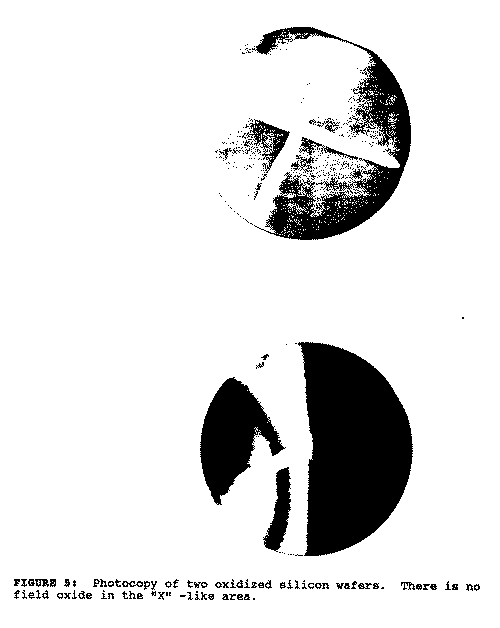I.4  Experimental Data Curves:

The wafer which was not coated was placed on the fixture and a baseline trace was made, ranging from 200 to 1,000 nm.  This is shown in Figure 6.  Then the oxidized wafer was traced.  The trace is shown in Figure 7 and the test of the nitride-coated wafer is shown in Figure 8.  Before delving into calculations and manipulations of the data, a "big picture" view of the data can be obtained by theoretical curves for the same physical conditions.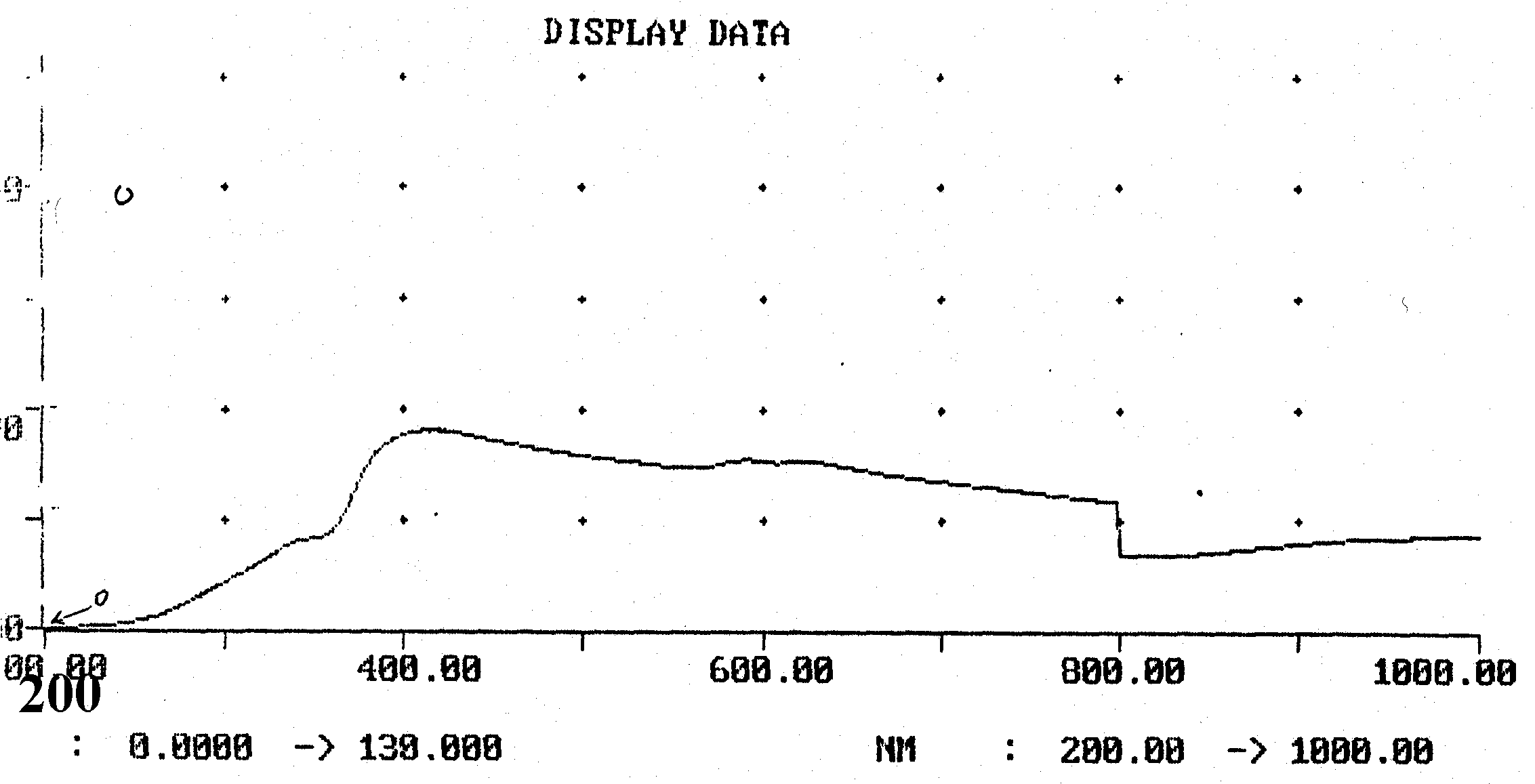FIGURE 6:  A baseline spectrophotometer trace.  A "bare" silicon wafer was placed on the reflectometry adaptor and this reference trace was made.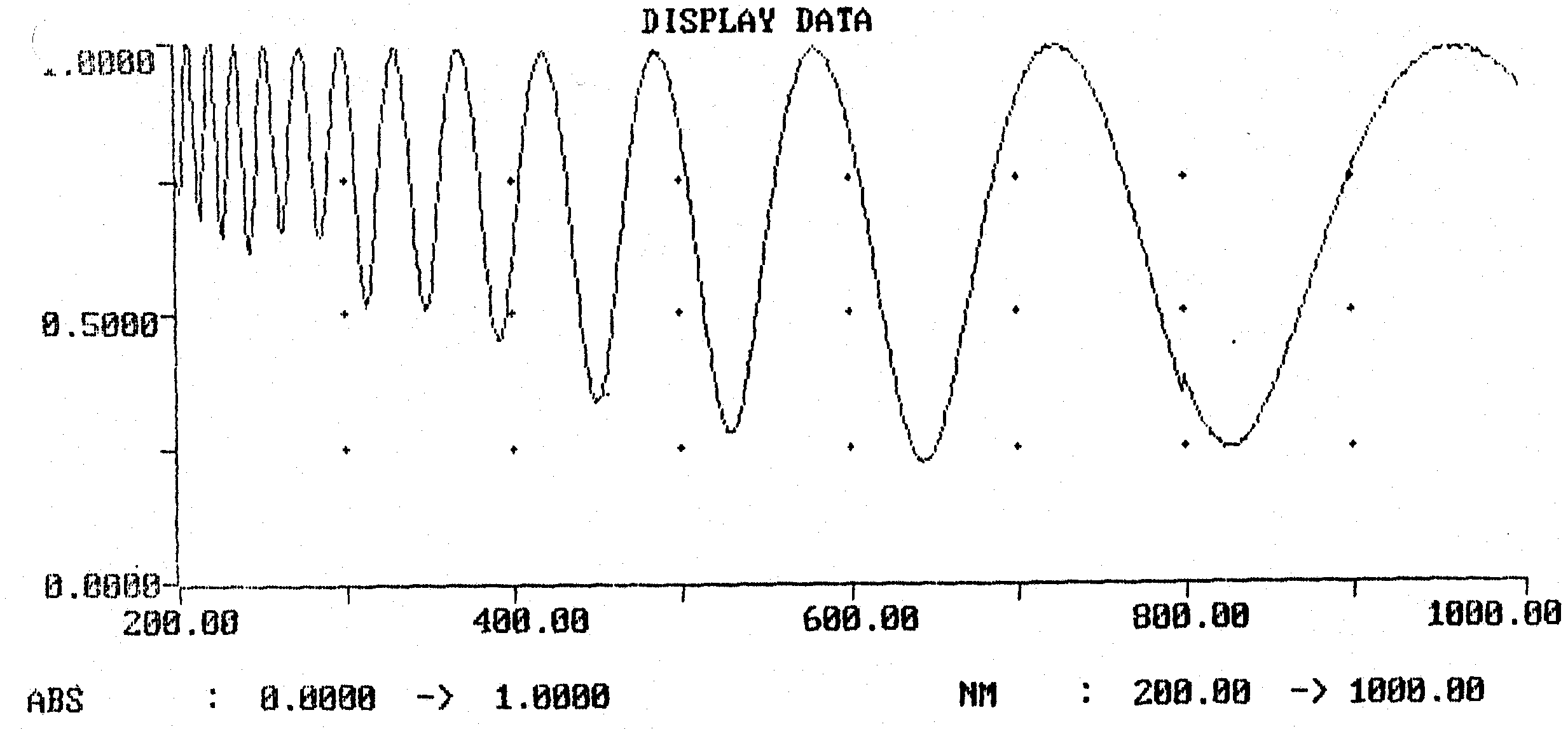FIGURE 7:  Spectrophotometry trace of reflection from an oxidized silicon wafer.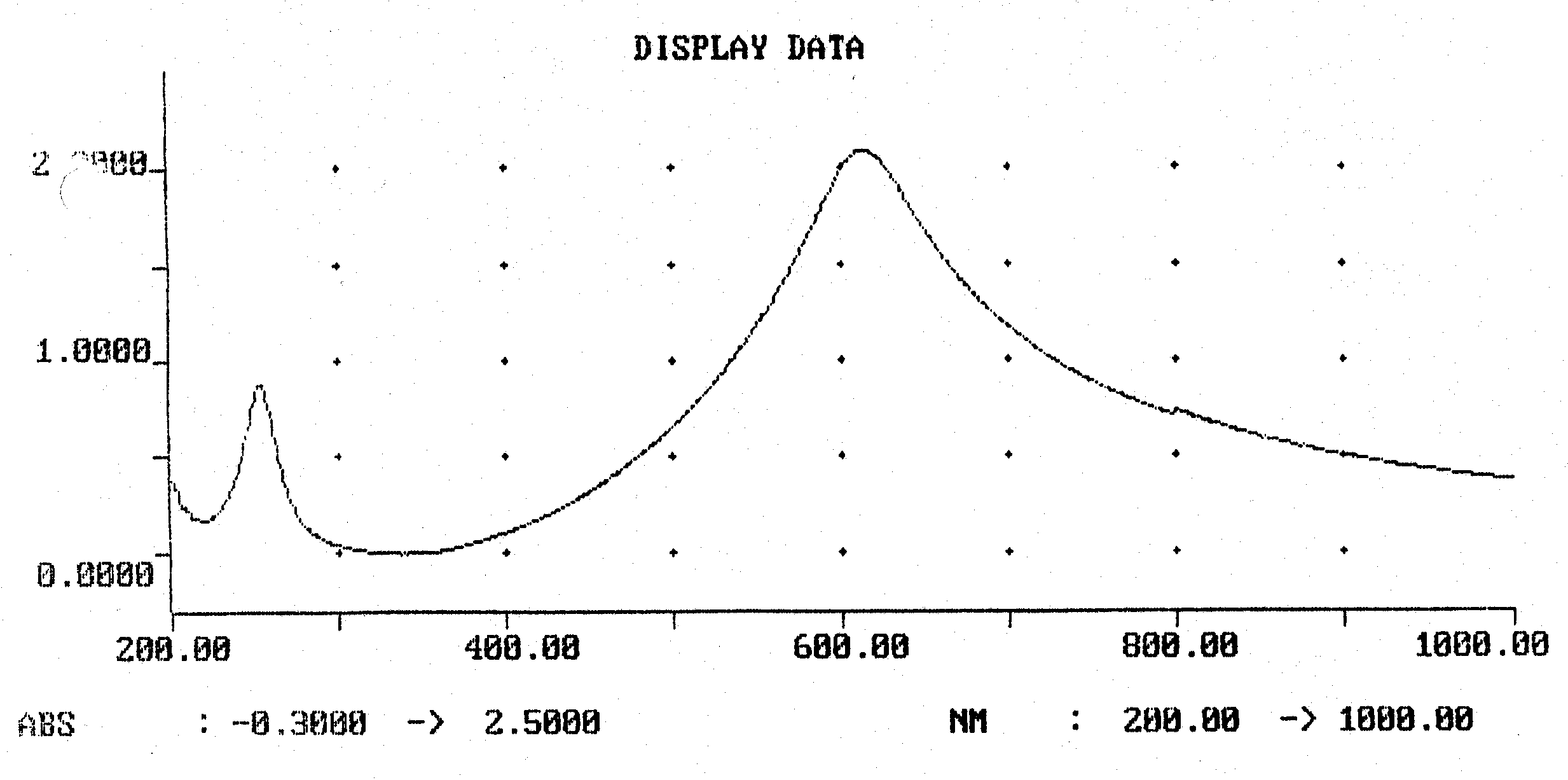FIGURE 8:  Spectrophotometry trace of reflection from a nitride coated silicon wafer.

I.5  Theoretical Amplitude Curves:

"Mathcad" software , was used to plot the theoretical curves.  The reflected amplitudes of radiation with electric field oscillations perpendicular and parallel to the incidence plane (Rs and Rp) were calculated via a formula which appeared as follows: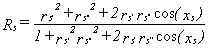2

where r's is the reflection (of perpendicularly polarized light) from the air to dielectric interface, r''s is the reflection from the dielectric-metal interface, and xs is the phase change caused by the optical path length travelled into and out of the film plus the phase change caused by the reflection from the metal surface δs: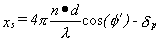3

Note r's and r'p, r''s and r''p, δs and δp, and Rs and Rp, are different for a non-zero angle of incidence.  Therefore, another major difference between this type of reflection measurements and typical transmission spectrophotometry is the difference in the traces of polarization components.

The thickness and index of refraction of the dielectric films were determined, for these theoretical plots, from ellipsometry measurements (to be discussed in the next chapter).  The cleanroom ellipsometer uses a beam laser with a wavelength of 623.8 nm.  Therefore, the value of n determined by ellipsometry is only good for that wavelength.  Since the values of n as a function of λ are required across the range of λ used in theoretical calculations, the Cauchy dispersion equation was assumed: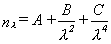4

where A, B, and C are constants.  These constants were approximately determined, by using the index value from ellipsometry (n632.8) and the indices at various wavelengths given in Table I for vitreous silica .  (The same was done for the nitride so that only the value of A differs from nitride and silica input.)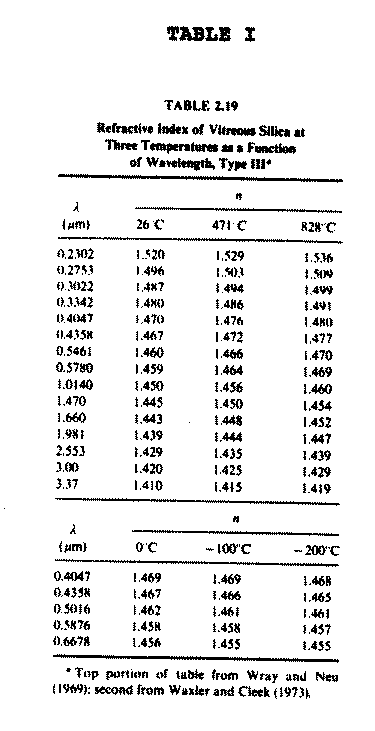TABLE I:  Index of refraction values for vitreous silica.  This data was used, along with ellipsometry data, to generate constants for the Cauchy dispersion equation.  (A = 1.416;  B = 4,000; C = 1.533 * 107)  Table taken from N. P. Bansal and R. H. Doremus, Handbook of Glass Properties, Academic Press, New York, 1986.

Other data required for generation of theoretical curves is the complex index of refraction for silicon (= ns + ks*i).  Both the real and imaginary (ns and ks) parts of the index vary greatly over the wavelength range used, as illustrated by the plot of ns and ks versus λ shown in Figure 9.  The data used for complex index was obtained from Aspnes and Theeten [4,5] and is shown in Table II.  The mistake was made of entering complex index values at intervals of only 1 nm and the software limited the quantity of inputs.  Therefore, theoretical calculations are only made for λ = 200 to 799 nm by 1 and not λ from 200 to 1,000 nm as in the experimental work.  However, the 200 to 799 nm range is broad enough to yield understanding of the theory.  Lastly, the complex index of refraction is slightly dependent on angle of incidence, but test calculation showed variations of only tenths of a percent, thus no attempt is made to correct for the different angles of incidence in this study.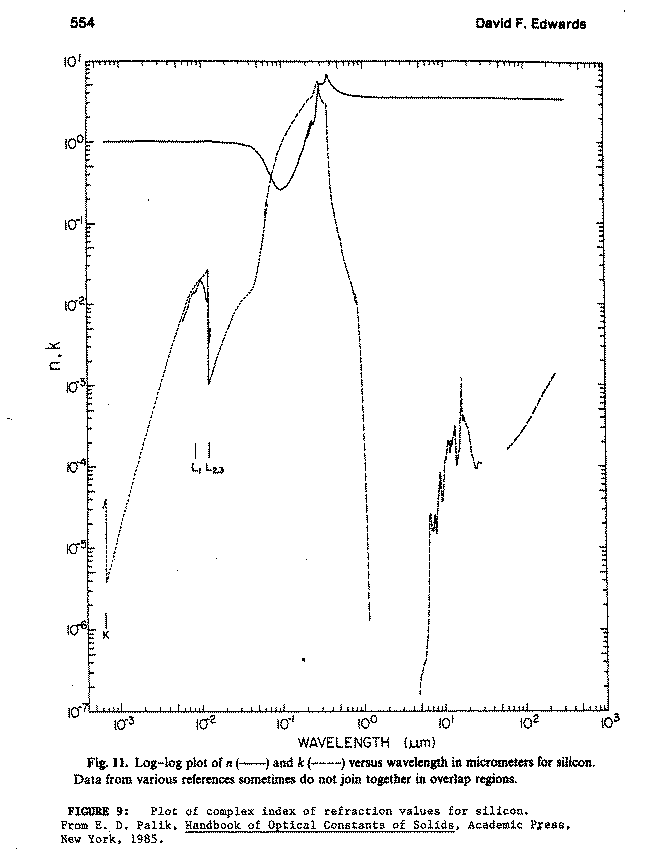The Mathcad work is shown here.  Figures 10a and 11 show three theoretical curves plotted on the Y-axis:  Rs/Rbs is the ratio of the amplitude of the perpendicular reflected component from the film covered substrate over the amplitude of perpendicular reflected component which would be reflected from the bare substrate.  Therefore, Rbs is a theoretical baseline for the perpendicular component.  Similarly, Rp/Rbp is plotted for the parallel components.  Finally, (Rs + Rp)/(Rbs + bp) is the ratio of total radiation amplitude reflected versus the total baseline (radiation reflected off "bare" silicon).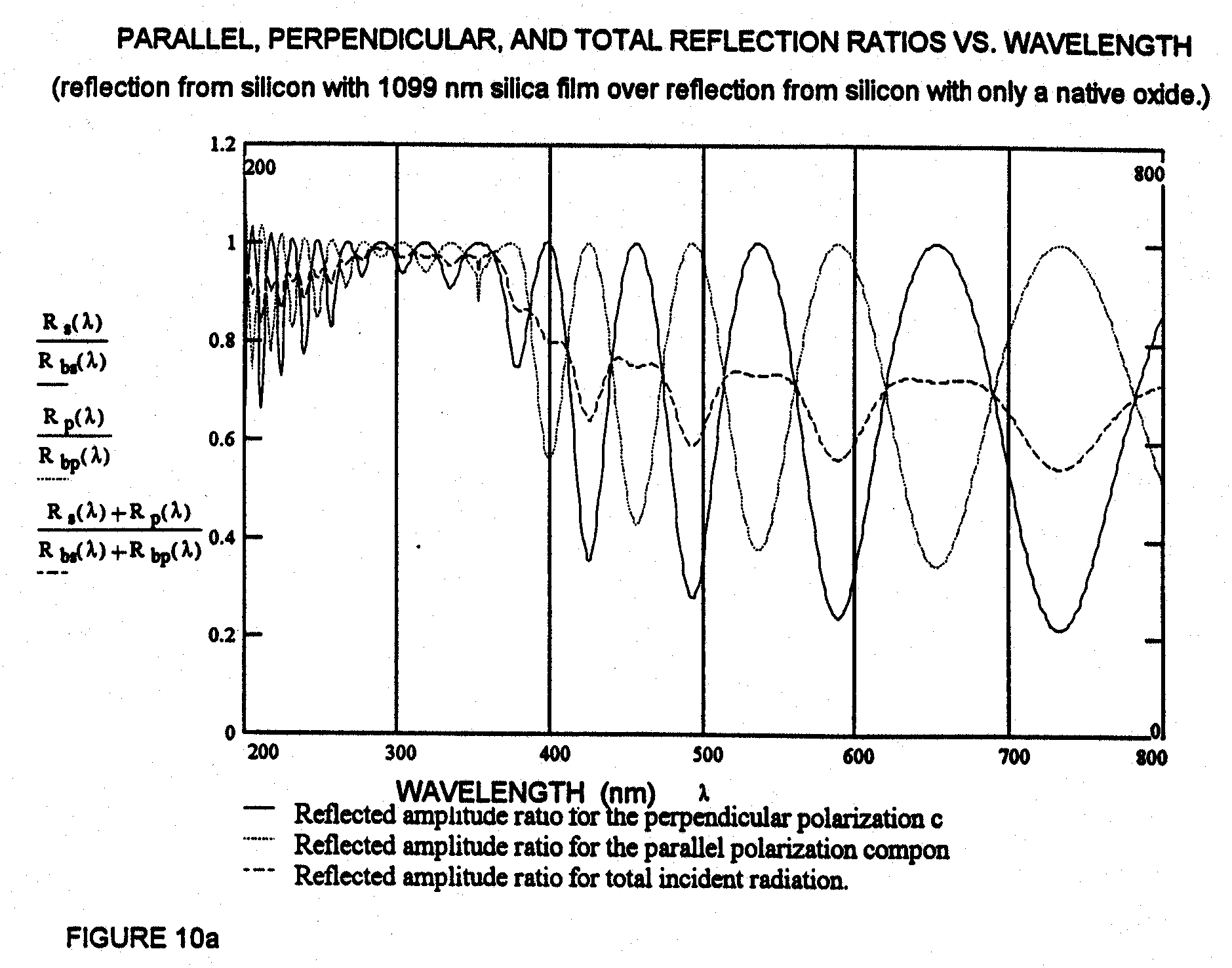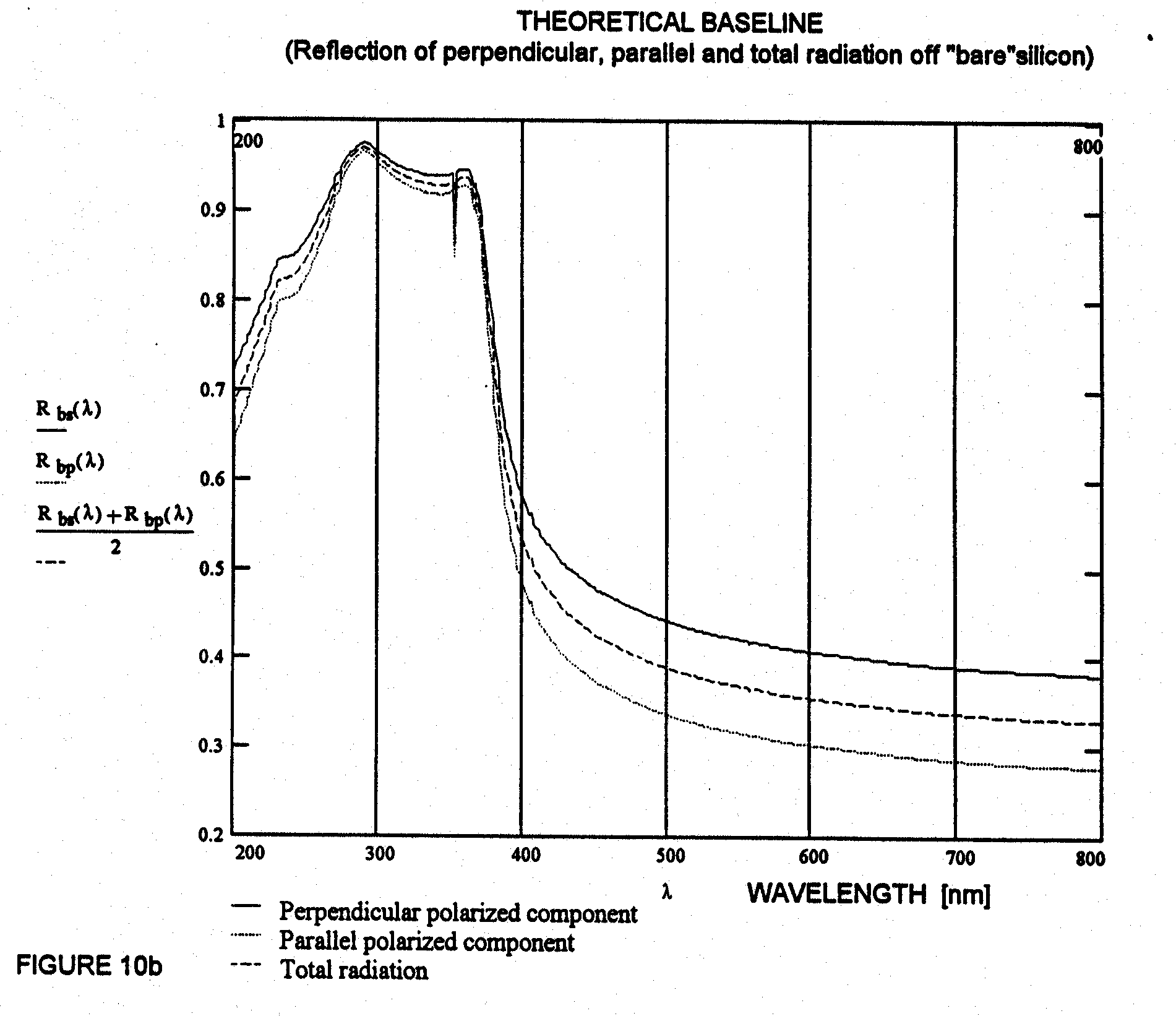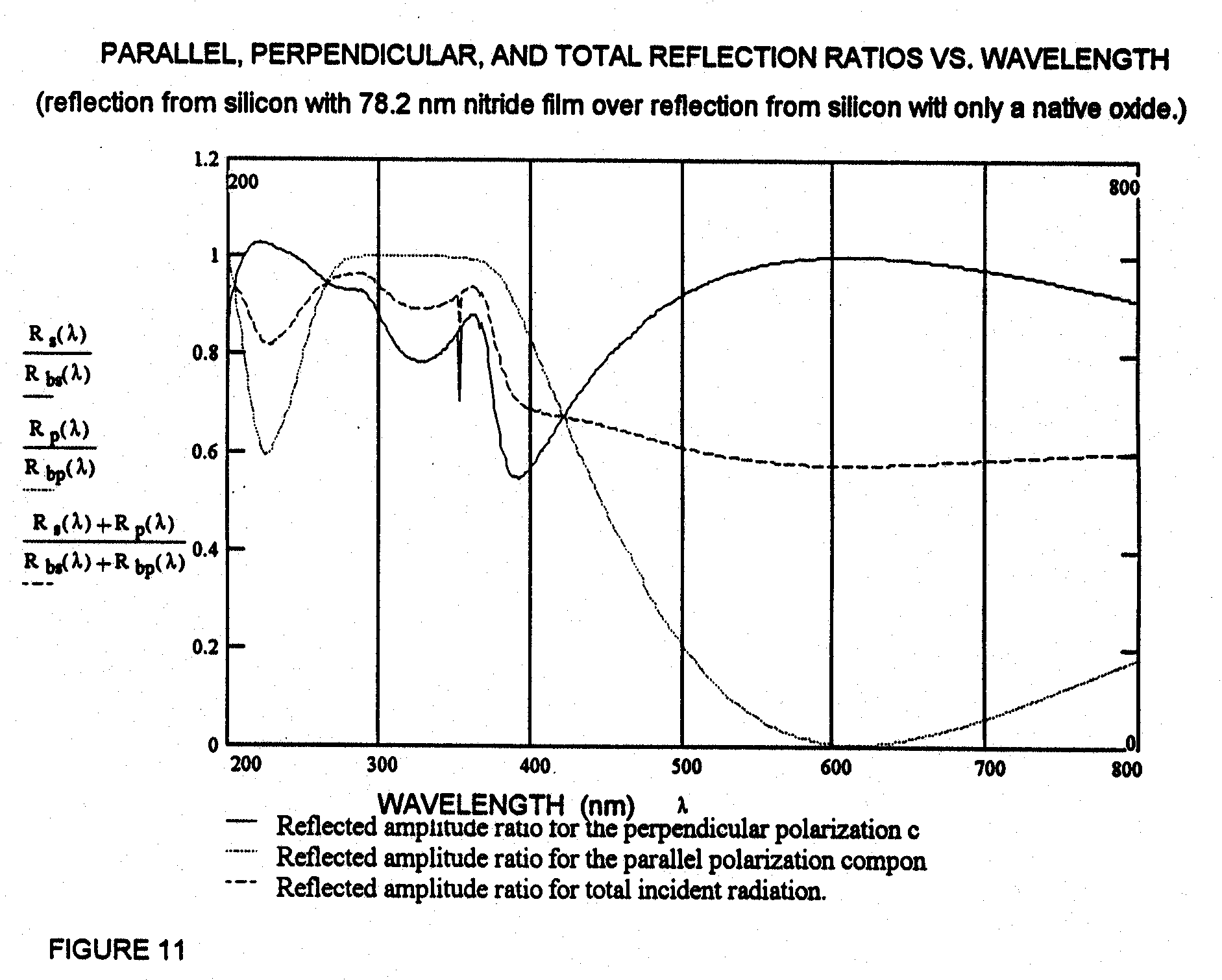The first thing that one notices is that the sinusoidal nature of the theoretical curves for the two polarization components matches the sinusoidal nature of the experimental curve.  But when the two component curves are averaged to determine the total reflected amplitude ratio, the curve cannot be considered to be sinusoidal.  It would seem that the experimental curve would be predicted by the averaged theoretical curve, because no polarizer was purposely placed in the system.  However, this is clearly not the case.  Therefore, the Cary 5's optical elements (slits, diffraction gratings, etc.) must cause significant polarization of the light beam.

It is noticed that the maxima and minima of the experimental curve (Figure 7) match the maxima and minima of the amplitude curve for the parallel polarization component exactly.  This indicates first that accurate n*d values will be determinable from the experimental data.  Secondly, it indicates that the perpendicular polarization component has been significantly reduced in the optical system.  This is easily proven by placing a polarizer in the system and performing baseline traces (this test has not been performed at this time).

The second difference is seen as the sharp reduction in oscillation amplitude for λ's below about 370 nm in the theoretical curve.   The experimental curve (Figure 7) retains significant oscillation amplitudes in that range.  The reason for this is easily seen by comparing the experimental baseline (Figure 6) to the theoretical baseline (Figure 10b).  The experimental reflection off silicon decreases sharply for λ's below 370 nm, while the theoretical reflection increases greatly below 370 nm.  Ignoring the native oxide, the reflection off the silicon is only dependent upon the complex index of refraction of silicon.   This indicates how dramatically the complex index can changes in this wavelength range, especially if the silicon is doped differently.  The silicon used by Aspnes and Theeten  had a resistivity of 26 Ω cm.  The resistivity of the wafers used in this study was not determined, but could be determined in the future.

Something that is not as easily seen is that the peaks of the theoretical curve exceed unity by a very small percentage.  And the measurement maximums from the experimental curve are less than one by a very small percentage.  This indicates two things:  1. the light is highly polarized, if it were not the experiment curve would not be as close to one because the parallel polarization component reaches a minimum at nearly the same λ where the p-component is maximum, and 2. the system is accurate (errors with this method are insignificant).

Before comparing the experimental and theoretical curves for the nitride layer, it will be noted that the ellipsometric data for the nitride revealed a film thickness of only 78.2 nm.  This means that there will be very few maxima and minima occurring over the wavelength range at which n*d values can be determined.  In comparing the experimental curve (Figure 8) with the theoretical curve (Figure 11), it can be seen that the two peaks in experimental curve correspond to the two peaks of the theoretical amplitude of the perpendicular polarization component (this will be discussed later).

The larger peak (occurring at 612 nm in the experimental curve) corresponds closely to the theoretical maximum of the s-component.  But the experimental peak is sharper and much higher (over 2 where the theoretical peak is only slightly above 1).  The experimental peak at the smaller wavelengths occurs at a larger wavelength than the theoretical, it is also sharper, but in this case it is smaller than the theoretical.  There appears to be a third peak at, about λ = 360 nm, in the theoretical curve.  But comparison of the shape of this peak with the theoretical baseline plot in Figure 10b, indicates that this is simply an influence from the varying index of silicon data used.

Why is there such differences between the theoretical and experimental for the nitride film, when the comparison for the silica was so close?  And, if the light beam is heavily polarized in the parallel direction, as was concluded from the previous comparison, why does the experimental curve match the perpendicular component in this case?  The answer is that the sample actually contains a double film on the silicon.  Since the nitride layer is so thin and since nitride has a much higher index than silicon dioxide, the native silicon dioxide layer has significant influence in this case.

Before answering the second question on polarization, it will be noted that the theoretical amplitude curves for the two polarization components are nearly π (180o) out of phase with each other.  This will occur when δs is very close in value to δp, which is true for the majority of the wavelength range used, and the incident angle is within a certain range (0 < φ < θp).  To demonstrate that this is valid consider the following case:  Assume that δs - δp = 0.  At the angles used in this study, light waves normal to the plane of incidence will experience a phase change of π when reflected at a boundary where the incident medium is less dense than transmitting medium and the normal waves will experience no phase change when reflected in a denser, incident medium.  The exact opposite occurs for waves parallel to the plane of incidence.  For parallel waves, there is no phase change from reflection where the incident medium is less dense and a π phase difference with a denser incident medium.  Since the parallel and perpendicular waves are compete opposites, it is easy to see that reflection at a metal with one thin film (where δs - δp = 0) will cause the maximum for one component to occur at the same wavelength of a minimum for the other component, and so on.  Therefore, it is fortunate that the Cary 5 inadvertently polarizes the measurement beam, otherwise the data would have been useless.

If another film is added to create a double film stack on silicon (and another interface), the p- and s- components would reverse.  In other words, the peaks would still be out of phase by π, but each component would have changed phase by π.  Therefore, Figure 11 indicates that the perpendicular component matches the experiment, because Figure 11 was generated for one film.  But it is actually the parallel component that matches.

I.6  Experimental Data:

The Cary 5's program allows for accurate determination of X and Y coordinates from a trace.  Therefore, these coordinates were determined for the plots in Figures 7 and 8, for the silica and nitride films.  The coordinates yield the wavelength values where a maxima or a minima occurs (X-axis) and Y-axis values, the ratio of reflected to baseline amplitudes.  Since, this system will not be able to determine n and d independently, a film index determined by ellipsometry was used initially.  This value is n632.8 = 1.426 for silicon dioxide and n632.8 = 1.997 for silicon nitride.  This is the index of refraction at the wavelength of the laser used with the ellipsometer.  This n632.8 is used to obtain a value for d using a maxima or minima nearest to λ = 632.8.  Then this value of d is used to determine the value of nλ at all the other maxima and minima.

It was noted earlier that the experiment data for the silica film case closely matched the theoretical curve for the parallel polarization component.  Equation 3 is the main equation used: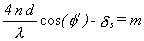(3)

where δp is the phase change a wave front experiences when reflected at the silica-silicon interface.  It is easier to calculate δp, by calculating Δ and δs: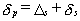6

and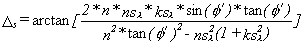7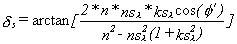8

where nsλ and ksλ are the real and imaginary parts of the complex index of refraction for silicon, as they vary with λ.  Since δp is dependent on n, n is determined for each λ by iteration.

For the silica film, using n632.8, a d value of 1082 nanometers was determined.  Also the λ values and the calculated n values, for the silicon dioxide film are listed in Table III.  The calculated index values in Table III were plotted in Figure 14 and a curve was fit to the data assuming the form of Equation 4, the Cauchy dispersion equation.  The maximum deviation of the experimental points from the fit curve was 0.6% and the standard deviation of the point from the curve was 0.3%.  Therefore, this technique can determine film thicknesses to plus or minus half a percent.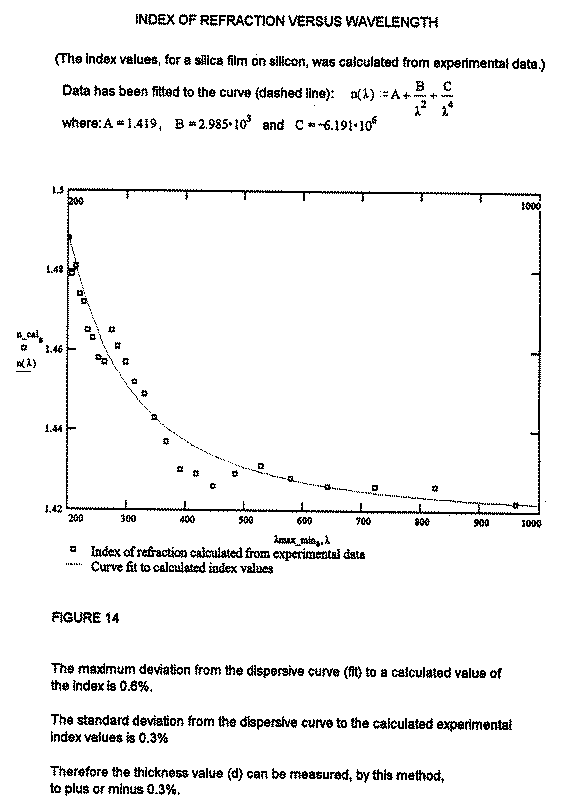FIGURE 14:  Index of Refraction versus Wavelength.

For the silicon nitride film the second dielectric layer will be ignored at this time.  Only two maxima were used (the minimum appears to be ambiguous).  With the nitride, δs was used in Equation 5 instead of δp, as discussed before.  δs is determined directly from Equation 7.  The largest maximum occurs at λ = 612, so using n = 1.998 (corresponding to n632.8 = 1.997) a nitride thickness of d = 76.6 nanometers was arrived at.  The value of n254 was then determined, for the other maxima at λ = 254 nm, to be n254 = 2.052.

TABLE III

Experimental and Calculated Data for the Silica Film

*Y-axis values are referenced to reflection from "bare" silicon.

**Index for the silica film is calculated using the λ values at listed & using an ellipsometer value (n632.8=1.426) to set d=1082 nm.

 λ [nm] Reflection      Ratio* Maxima or Minima Calculated Index (n)** 961 0.9836 MAX 1.422 826 0.2464 MIN 1.426 723 0.9885 MAX 1.426 643 0.2191 MIN 1.426 580 0.9880 MAX 1.428 529 0.2764 MIN 1.431 485 0.9839 MAX 1.429 448 0.3349 MIN 1.426 418 0.9841 MAX 1.429 392 0.4498 MIN 1.430 368 0.9867 MAX 1.437 348 0.5055 MIN 1.443 330 0.9879 MAX 1.449 313 0.5123 MIN 1.452 298 0.9983 MAX 1.457 285 0.6428 MIN 1.461 274 0.9904 MAX 1.465 262 0.6548 MIN 1.457 252 0.9941 MAX 1.458 243 0.6118 MIN 1.463 234 1.0019 MAX 1.465 227 0.6435 MIN 1.472 220 1.0009 MAX 1.474 214 0.6774 MIN 1.481 207 1.0007 MAX 1.479 202 0.7261 MIN 1.488

TABLE IV

Experimental and Calculated Data for the Silicon Nitride Film

*Y-axis values are the ratio of reflection from the sample to reflection from a "bare" silicon wafer used as a reference.

**Index for the silica film is calculated using the λ values at listed and using an ellipsometer value (n632.8=1.997), which allowed determination of d = 76.6 nm.

 λ [nm] Reflection      Ratio* Maxima or Minima Calculated Index (n)** 612 2.09 MAX 1.997 254 0.88 MAX 2.052

This index value is well within the range of possible indices due to dispersion.  Therefore, it is again concluded that this technique can determine film thicknesses accurately.

I.7  Suggested Improvements:

So far the system has proved to be ruggedly accurate for the determination of the product of n*d.  However, it is desirable to determine n and d independently.  It is obviously redundant to use the ellipsometry to determine a value of n in practice, as it was determined in this study.  Furthermore, using a textbook value for n can yield significant errors in d.  This can be seen by comparing the experimental and textbook values of n for silica shown in Tables III and I respectively.  There is over a two percent difference in n from experimental and textbook.

The suggested method for measuring n and d is to start with a test beam which is not significantly polarized and make two traces, one for p-polarized then turn the polarizer 90o for s-polarized.  This requires four scans altogether (two baselines and two traces).  This would essential be a spectrophotomic ellipsometer and thus would be more accurate, versatile, and capable than a single λ ellipsometer.  The use of ellipsometry is will be described further in the next chapter.

See our Surface Oxide Tester, which  measures the film thickness' on copper in Angstroms with the speed and user-convenience required in QC Test Labs.

Home